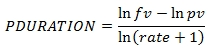# SQL Server Period Duration function

PDURATION
Updated: 5 Apr 2017

Use the scalar function PDURATION to calculate the number of periods required by an investment to reach a specified value. The formula is:fv = the future value of the investment pv = the present value of the investment rate = the periodic interest rate.
Syntax
SELECT [wct].[PDURATION] (
<@Rate, float,>
,<@PV, float,>
,<@FV, float,>)
Arguments
 Input Name Description @Rate Periodic interest rate. @Rate must be of type float or of a type that implicitly converts to float. @PV Present value of the investment. @PV must be of type float or of a type that implicitly converts to float. @FV Future value of the investment. @FV must of a type float or of a type that implicitly converts to float.
Return Type
float
Remarks
• If @PV is NULL then @PV = 0
• If @PV = 0 then NULL is returned
• If @FV is NULL then @FV = 0
• If @FV = 0 then NULL is returned
• If @Rate is NULL then @Rate = 0
• If @Rate = 0 then NULL is returned
• If @Rate < -1 then NULL is returned
• Available in XLeratorDB / financial 2008 only
Example
SELECT
wct.PDURATION(
wct.PERIODRATE(.08,1,12)/12    --@Rate
,1000        --@PV
,1259.712    --@FV
) as PDURATION

This produces the following result.Copyright 2008-2021 Westclintech LLC         Privacy Policy        Terms of Service